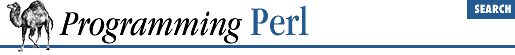home | O'Reilly's CD bookshelfs | FreeBSD | Linux | Cisco | Cisco Exam

#### 3.2.101 oct

```oct ```

EXPR
```
```

This function interprets ``` EXPR ``` as an octal string and returns the equivalent decimal value. (If ``` EXPR ``` happens to start off with ``` 0x``` , it is interpreted as a hex string instead.) The following will handle decimal, octal, and hex in the standard notation:

`\$val = oct \$val if \$val =~ /^0/;`

If ``` EXPR ``` is omitted, the function interprets \$_ . To perform the inverse function on octal numbers, use:

`\$oct_string = sprintf "%lo", \$number;`